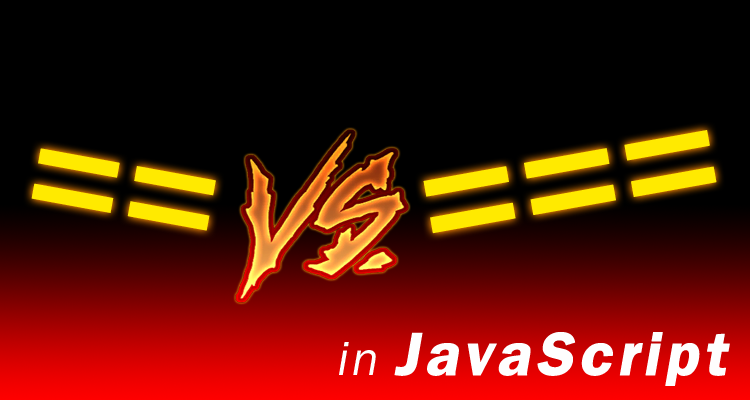# == vs. === in JavaScript: Equal vs. Kinda Sorta EqualA common explanation is that triple equals is more “strict” than double equal.

But that doesn’t tell you the whole story, it’s actually simpler and also more complicated than that.

For example did you know that the one with less equal signs is actually the one that does more.

It’s true. Triple equal does a straight up comparison, types have to be equal and values have to be equal, that’s it.

Double equal `==` on the other hand will actually change the values so that it can compare them.

That’s why it gets you into trouble, you think you know when it will it true or false, but you’ll be surprised.

Take a look, what do you think this code will do?

```'\r\n\t' == 0
```

I thought it was going to return `false` – but it actually returns `true`!

Don’t believe me see for yourself:

https://jsfiddle.net/luisperezphd/90v093fy/

```a = '' // empty string
b = '0' // string literal of the number zero
c = 0 // the actual number 0
```

Well if `a == c`, which it does, it returns `true`.
And `b == c`, which is also `true`

Then `a == b` must be true, right?

Nope!

That’s not even the worse of it, I have some examples you won’t believe.

Before I show you those though, you know how I said that the double equal actually does more work than the triple equals.

It’s true, in fact you could actually write a function that does exactly what `==` using “strict” operators like `===`.

Here’s the function to prove it:

```// loseEqual() behaves just like `==`
function loseEqual(x, y) {
// notice the function only uses "strict" operators
// like `===` and `!==` to do comparisons

if(typeof y === typeof x) return y === x;

if(typeof y === "function" || typeof x === "function") return false;

// treat null and undefined the same
var xIsNothing = (y === undefined) || (y === null);
var yIsNothing = (x === undefined) || (x === null);

if(xIsNothing || yIsNothing) return (xIsNothing && yIsNothing);

if(typeof x === "object") x = toPrimitive(x);
if(typeof y === "object") y = toPrimitive(y);

if(typeof y === typeof x) return y === x;

// convert x and y into numbers if they are not already use the "+" trick
if(typeof x !== "number") x = +x;
if(typeof y !== "number") y = +y;

return x === y;
}

function toPrimitive(obj) {
var value = obj.valueOf();
if(obj !== value) return value;
return obj.toString();
}
```

This function should shed some light on why you always hear people say that you should be using triple equal `===` not double equal `==`.

It’s just double equal does a lot and it gets kinda complicated so it’s hard to know what to expect so you can easily end up with bugs.

The moral of the story is to use the “simpler” triple version of operators whenever possible, not just triple equals, but also `==`, and `<==`, so on and so on.

The results will be much more predictable.

Here are those other crazy examples I told you about:

(feels like the blooper reel of a TV show)

Inconvenient Truths

``` == true // returns true
'0' == false // returns true
[] == false // returns true
[[]] == false // returns true
 == false // returns true

[1,2,3] == '1,2,3' // returns true

'\r\n\t' == 0 // returns true
```

Unexpected Conclusions – aka Things that make you go Huh?

```// IF an empty string '' is equal to the number zero (0)
'' == 0 // return true

// AND the string zero '0' is equal to the number zero (0)
'0' == 0 // return true

// THEN an empty string must be equal to the string zero '0'
'' == '0' // returns **FALSE**
```

Objects with Special Functions – Weird Town

```// Below are examples of objects that
// implement `valueOf()` and `toString()`

var objTest = {
toString: function() {
return "test";
}
};

var obj100 = {
valueOf: function() {
return 100;
}
};

var objTest100 = {
toString: function() {
return "test";
},
valueOf: function() {
return 100;
}
};

objTest == "test" // returns true
obj100 == 100 // returns true
objTest100 == 100 // returns true

objTest100 == "test" // returns **FALSE**
```